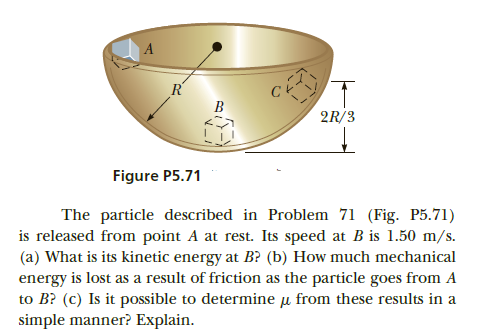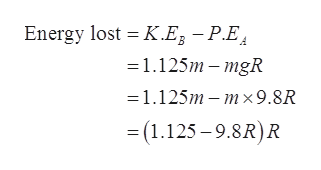# R'B2R/3Figure P5.71The particle described in Problem 71 (Fig. P5.71)is released from point A at rest. Its speed at B is 1.50 m/s.(a) What is its kinetic energy at B? (b) How much mechanicalenergy is lost as a result of friction as the particle goes from Ato B? (c) Is it possible to determine u from these results in asimple manner? Explain.

Question
13 viewshelp_outlineImage TranscriptioncloseR' B 2R/3 Figure P5.71 The particle described in Problem 71 (Fig. P5.71) is released from point A at rest. Its speed at B is 1.50 m/s. (a) What is its kinetic energy at B? (b) How much mechanical energy is lost as a result of friction as the particle goes from A to B? (c) Is it possible to determine u from these results in a simple manner? Explain. fullscreen
check_circle

Step 1

Given data:

Speed of the particle at A, VA = 0 m/s

Speed of the particle at B, VB = 1.50 m/s

Let the mass of the particle be m

Step 2

(a)

The kinetics energy of the block at B:

Step 3

(b)

The mechanical energy lost as the ...help_outlineImage TranscriptioncloseEnergy lost = K.E, – P.E. =1.125m – mgR =1.125m – m × 9.8R = (1.125 – 9.8R)R fullscreen

### Want to see the full answer?

See Solution

#### Want to see this answer and more?

Solutions are written by subject experts who are available 24/7. Questions are typically answered within 1 hour.*

See Solution
*Response times may vary by subject and question.
Tagged in

### Other